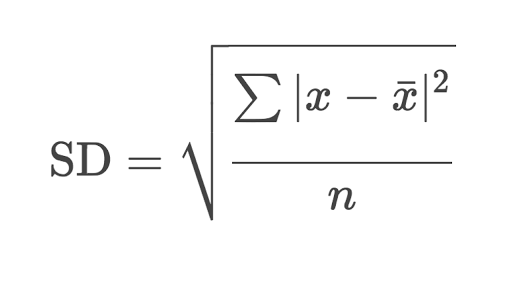# How to Calculate Standard Deviation in a smartsheet?

Hi, I'm working on getting all metrics for a large project into a dashboard in smartsheets. I've been tracking a burn up chart in google sheets, but think that connecting directly to it doesn't look clean. So in an attempt to copy it over, I'm starting from scratch but can't seem to find how to do standard deviation as a function. Is this available? Do I have to create it somehow?

Thanks,

PO

• you gotta build it out, but it shouldn't be that difficult. what is your column name for the data you want to find the standard deviation for?• https://app.smartsheet.com/b/publish?EQBCT=ca720c75a0da48c2a74f47f7cd537aba

Helper column formula when column name is A:

=(A1 - AVG(A:A)) ^ 2

STD DEV formula when helper column name is Helper:

=(SUM(Helper:Helper) / COUNT(A:A)) ^ 0.5

• Hi ProductOwner and L,

These may help:

Estimates standard deviation based on a sample, ignoring logical values and text.

Calculates standard deviation based on an entire population given as arguments, ignores logical values and text.

Estimates standard deviation based on a sample of a population.

STDEVPA(range, [additional_values])    Calculates standard deviation based on an entire population given as arguments, including logical values and text.

Best,

Kara

• Is it possible to run STDEVS for a specific vlookup range for a specific key elements, for a specific date range?

=STDEVS({RANGE},"Assignment1","Spring","2018")

• little late to the party but you should be able to use collect

stdevs(collect())

## Help Article Resources

Want to practice working with formulas directly in Smartsheet?

Check out the Formula Handbook template!